## Wednesday, December 13, 2017

### Personal evaluation. Data science MOOCs

Course Offered by Taken date Difficulty Commitment Type Description
Introduction to Data Science in Python Coursera. University of Michigan January 2017 ★★★☆☆ 10 hrs per week Theory and practice
Data Science in Real Life Coursera. Johns Hopkins University March 2017 ★☆☆☆☆ 3 hrs per week Theory and practice
A Crash Course in Data Science Coursera. Johns Hopkins University April 2017 ★☆☆☆☆ 3 hrs per week Theory A really introductory course. A lot of definitions about important concepts in data science. Any student should be able to pass this course
Data-driven astronomy Coursera. The University of Sydney April 2017 ★★★☆☆ 6 hrs per week Theory and practice An extremely fun course, really intuitive. Perfect for anyone interested in Astronomical data
Data processing using python Coursera. Nanjing University December 2017 ★☆☆☆☆ 4 hrs per week Practice An easy course. However, it is in Chinese and reading the subtitles is extremely tiring
Python data representations Coursera. Rice University December 2017 ★☆☆☆☆ 4-5 hrs per week Practice An very interesting course, the lectures are great. Very good professors.
Introduction to Structured Query Language (SQL) Coursera. University of Michigan December 2017 ★☆☆☆☆ 2-3 hrs per week Practice One of the best professors. A simple but great introductory course to sql
SQL for Data Science) Coursera. University of California, Davis January 2018 ★☆☆☆☆ 4 hrs per week Theory & Practice A really great way to learn SQL. It is an introductory course but it covers more aspects than a similar course from U. Michigan. If you are completely new to SQL i recommend to take first the one from U. Michigan
An Intuitive Introduction to Probability Coursera. University of Zurich January 2018 ★☆☆☆☆ 3-4 hrs per week Theory and Practice A very clear introduction to probability. The professors introduce the theory with interesting examples.
Python Data Analysis Coursera. Rice University February 2018 ★★☆☆☆ 4-5 hrs per week Practice This is an easy course if you are familiar with nested dictionaries in Python, otherwise it will be difficult at the beginning of the course
Python Data Visualization Coursera. Rice University March 2018 ★★☆☆☆ 4-5 hrs per week Practice This is the second part of the course Python Data Analysis; thus, if you are comfortable with dictionaries you should be fine

## Saturday, June 4, 2016

### Chasing outliers

Chasing outliers
The identification of outliers is a crucial step in almost any data analysis project. These outliers can be seen as deviation from the normal behavior , errors, surprises, etc, being their identity completely application dependent; despite their multiple and dependable identity, their proper identification can provide useful hints to decide if they should be identified as valuable information, ignored or modified to have a better fit with the rest of the data. However, before initiating any quest, it is important to consider the specific characteristics of the type of outlier we are searching for and the context where they are located.
One of the simplest types of outliers is that known as point outliers. The most basic example of this type are the values found at the extremes of a series of points. In R it is really simply to identify these outliers using the function outlier in the library outliers. However, for now we will identify them without using a specific library, instead we will use the basic and simplistic rule of 3 standard deviations ($${\sigma}$$) above the mean (Figure 1). Then, any observations lying beyond 3$${\sigma}$$ is declared as an outlier.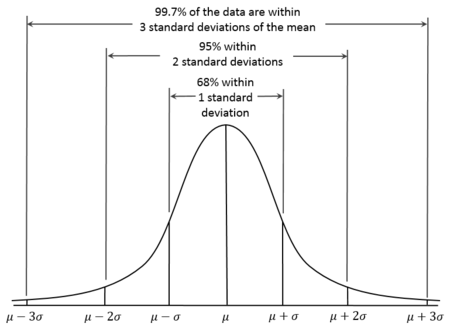Figure 1. The normal distributionhttps://en.wikipedia.org/wiki/68%E2%80%9395%E2%80%9399.7_rule”.
We’ve simulated a very small set of values(n=32) with only a pair of outliers:
##   0.72 0.88 1.78 1.04 1.06 1.86 1.23 0.37 0.66 0.78 1.61 1.18 1.20 1.06
##  0.72 1.89 1.25 0.02 1.35 0.76 0.47 0.89 0.49 0.64 0.69 0.16 1.42 1.08
##  0.43 1.63 7.21 6.85
In this small and, lets face it, unreal example the outliers 7.21 and 6.85 are located in a single dimensional dataset. Then, it is pretty easy to find them visually with the aid of a boxplot, on examination of Figure 2 it is evident that there is a pair of outliers(blue circles above the upper Whisker) in the data.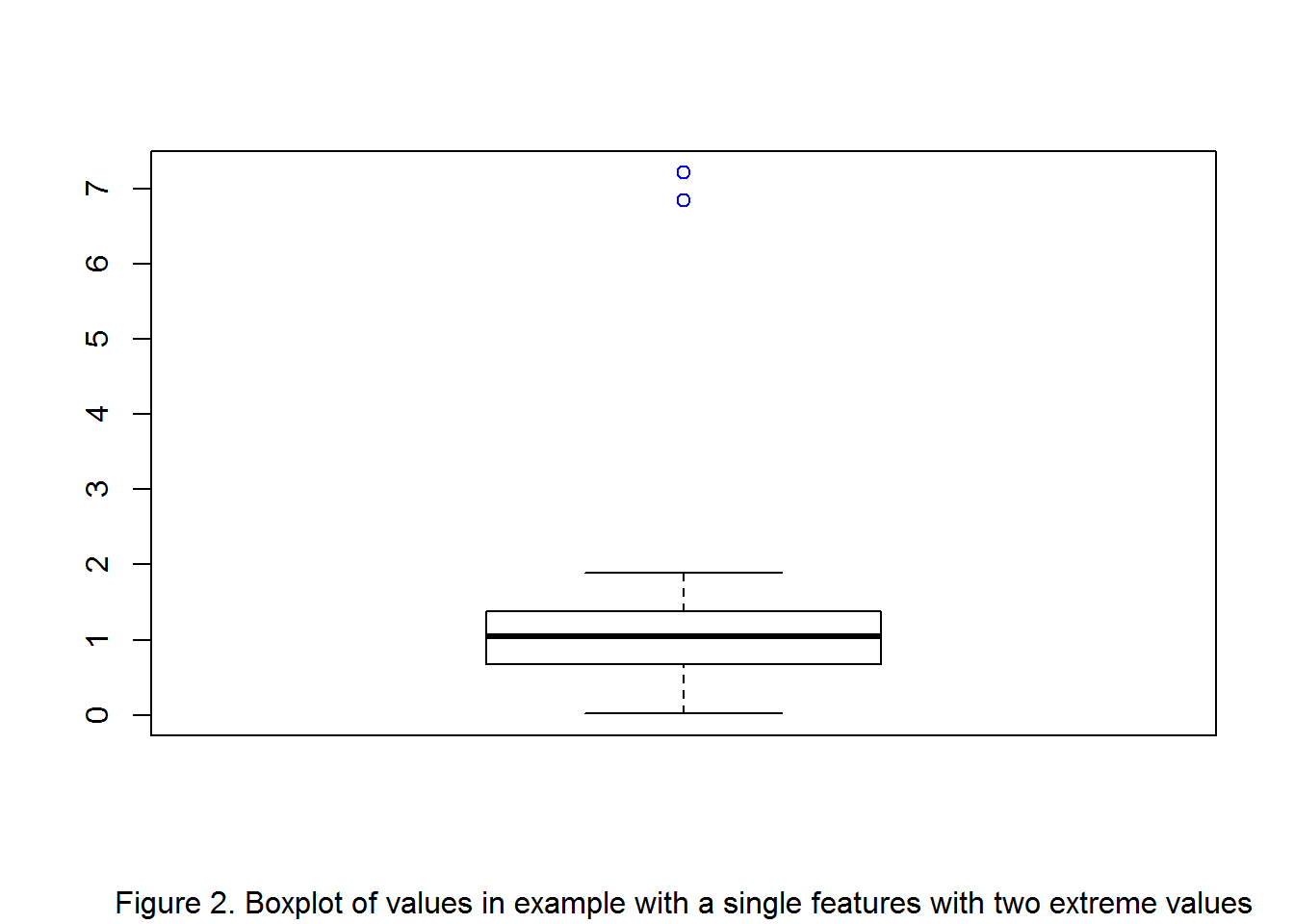With a single line of code it is possible to find them using the 3$${\sigma}$$ above the mean rule.
extreme_values<-small_example[which(small_example>mean(small_example)+(sd(small_example)*3))]
extreme_values
##  7.21 6.85
In this small and, lets face it, unreal example the outliers 7.21 and 6.85 are located in a single dimensional dataset; then, it was really easy to find them using the 3$${\sigma}$$ rule. However, in real world datasets the identification of outliers usually is done considering more complex scenarios, with multiple and interacting features, huge dimensionalities, etc.
Now, we will work in a more complex and realistic scenario where the outliers are located in more than a single dimension. With this purpose we’ve generated a two dimensional dataset. This artificially generated dataset is also far from real world scenarios where the dimensionality can reach millions of features, like in the genomic or the semantic analysis scenarios.However, despite its limitations this small dataset will suffice to explain a different way to search outliers. In Figure 3 we have plotted the dataset, clearly there are two clusters $$C_{1}$$ and $$C_{2}$$, the former has a very high density as its observations or points are very close to one another, the later being its points more sparsely distributed has a lower density. A problem with this type of scenario with different cluster densities is that it is not possible to use a simple algorithm like k-NN. k-NN could easily find the outlier $$O_{2}$$; however, k-NN can have problems to identify outlier $$O_{1}$$, due to the different densities of the clusters, possibly missing the outlier $$O_{1}$$ and instead classifying it as a normal or inlier observation.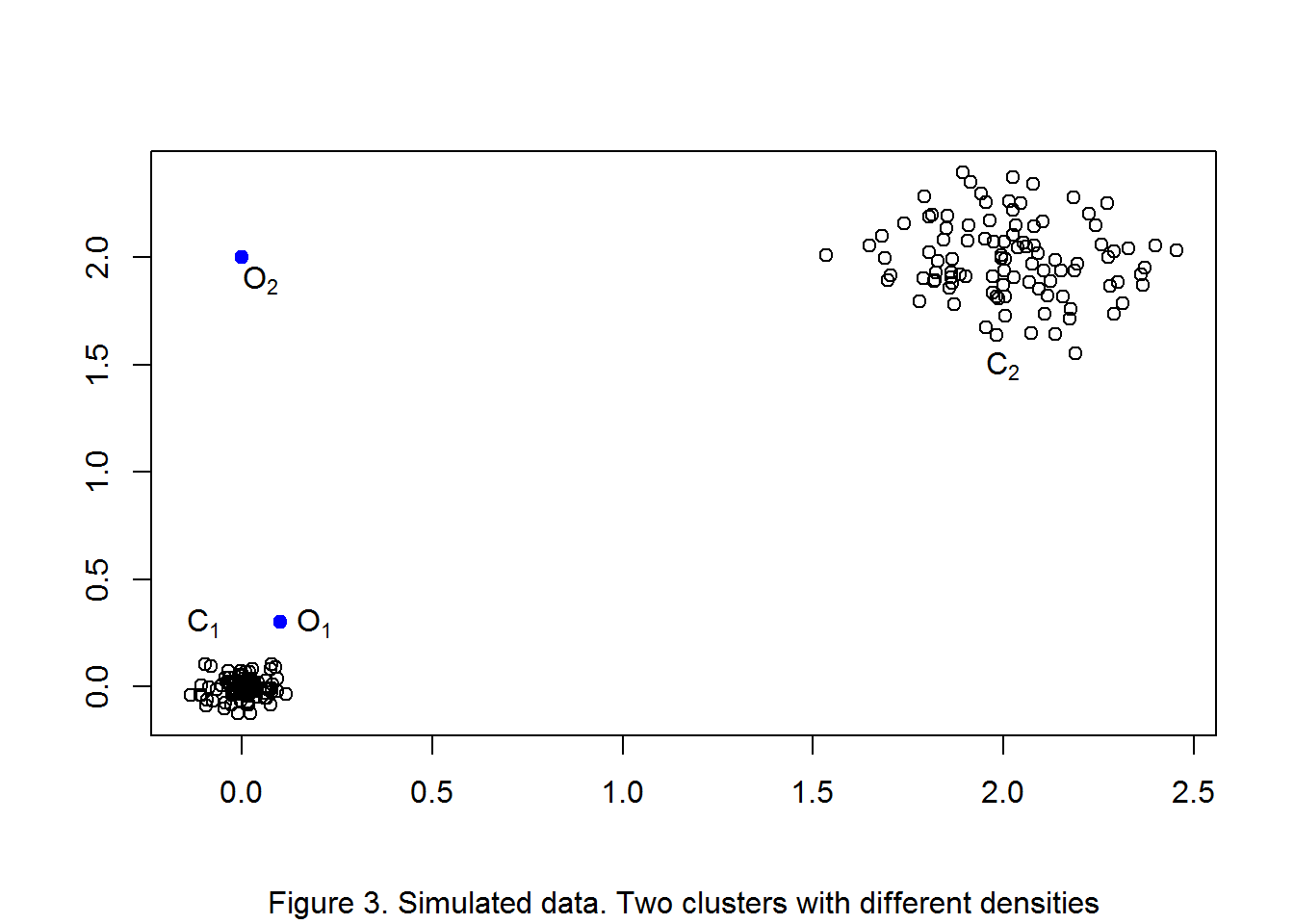In this type of scenario a better approach is to use a variation of k-NN known as Local Outlier Factor (LOF). LOF computes outlier scores by taking into account the different densities of each cluster and the distance to each neighbor. We can compute LOF outlier scores for each observation in the dataset using the function lofactor() in the DMwR library. The LOF function (Figure 3) takes only two parameters: the dataset and number of neighbors k; however, finding the best k depends on some factors like the size of the available sample, size of clusters, etc. These factors are completely application dependent, being then outside the limits of this post. We will arbitrarily fix k to a value of 5, but different values of k should be tested to find the best parameters for the specific dataset under study.
Let’s go over each component of the function lofactor()(Figure 4):
1. A variable to save the outlier score(one from each observation).
2. The function that actually computes the outlier scores.
3. The data.
4. the number of neighbors k.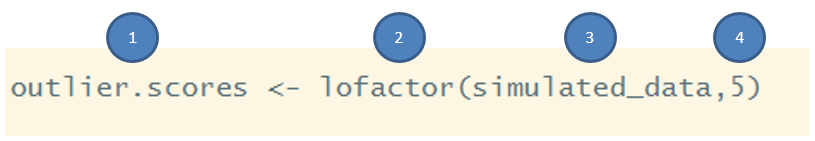Figure 4. LOF function.
Then, using the R DMwR implementation to compute outlier scores with LOF can be done with a single line of code, which produces a score for each observation in the dataset, the higher the score the higher LOF considers that observation to be an outlier, and the lower the score the higher LOF considers the observation to be a normal observation.
library(DMwR)
outlier.scores <- lofactor(data01,5)
On observation of the summary and density plot(Figure 5) of the scores generated by LOF it is clear that the distribution of scores is right skewed, with a mean and median of 1.2 and 1.0 respectively . Indeed, in this simplistic example LOF correctly assigned the highest scores to the two outlying observations, an oultier score of 9.14 to $$O_{2}$$ and 3.71 to $$O_{1}$$.
summary(outlier.scores)
##    Min. 1st Qu.  Median    Mean 3rd Qu.    Max.
##  0.9159  0.9901  1.0440  1.2290  1.1710  9.1460
plot(density(outlier.scores),sub="Figure 5. Outlier scores density")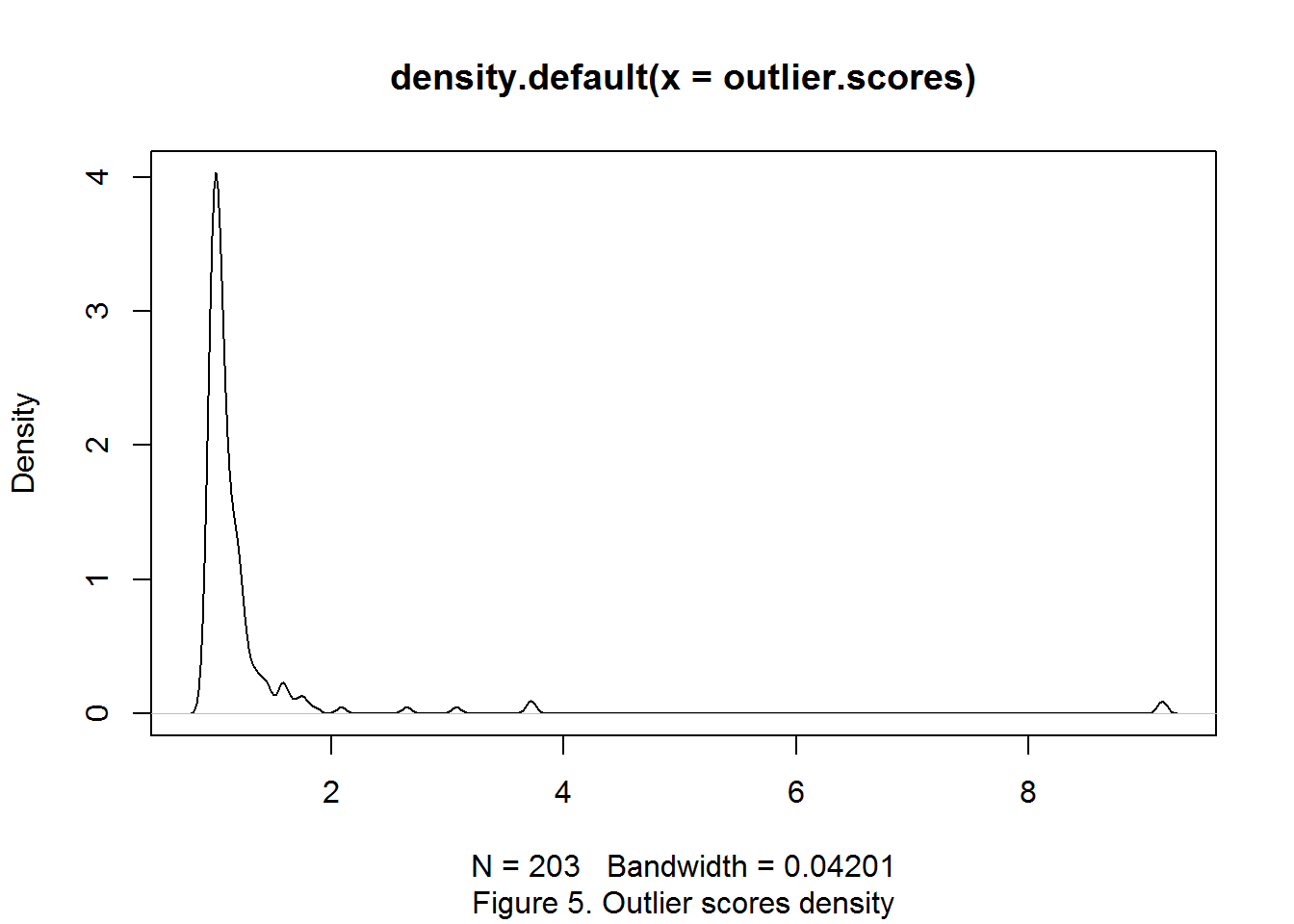It is worth noting that even an algorithm based on relative densities (or local densities) like LOF, can have some difficulties in differentiating between normal instances and outliers like $$O_{1}$$. Then, when tuning the algorithm parameters, data visualization, exploratory analysis and expert knowledge are key factors to obtain the best possible output, and different iterations of the algorithm need to be done before finding the sweet spot.
In this post we have explained two iconic approaches for outlier detection: the 3$${\sigma}$$ rule(mainly used for extreme value detection) and Local Outlier Factor (LOF). LOF is a very useful algorithm that can be used in real world scenarios, mainly due to its capacity to adapt to different local densities. However, many more algorithms for outlier detection exist in the literature 2, each of them based on different assumptions about what constitutes an outlier, being their use completely application dependent. Nevertheless, these algorithms have a common characteristic: their implicit design to find those observations exhibiting an aberrant behavior.